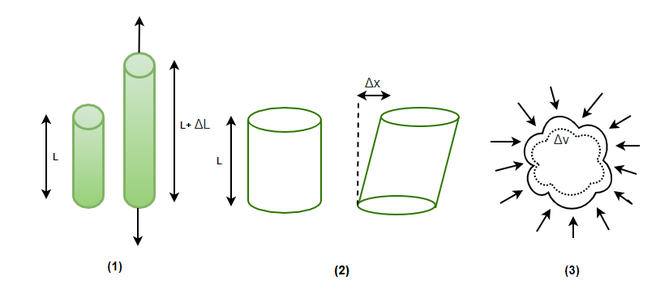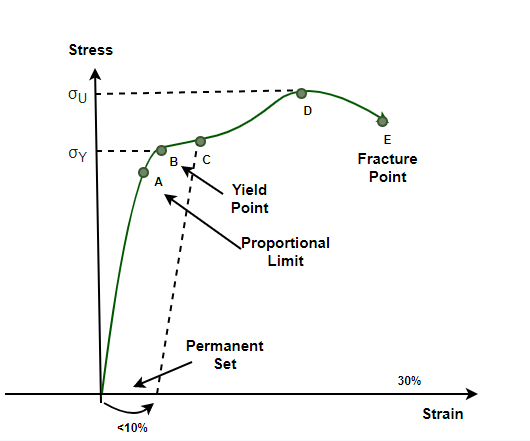GeeksforGeeks App
Open AppBrowser
Continue

## Related Articles

A solid is assumed to be a rigid body, but in real life, no body is perfectly rigid. All the solids change their shape when force is applied to them. For example, whenever a spring is compressed. The spring changes its size but comes back to its original position as soon as the force is removed. This property of solids is called elasticity and the deformation which is caused in this process is called elastic deformation. Some materials do not exhibit elastic properties. For example, if a force is applied to a puddle of mud, it just changes its shape without resistance. These bodies are called plastic and the property is called plasticity. Let’s study elastic bodies and elasticity in detail.

### Stress and Strain

When forces are applied to bodies that are elastic in nature, a temporary deformation is caused in them which depends on the nature of the material. This deformation is usually not visible, but it produces a restoring force that tends to bring back the body to its natural state. The magnitude of the restoring force is equal to the force that is applied to the body. Stress has been defined as the restoring force per unit area.

Let F be the magnitude of the force applied on the body and A be the area,

Stress =The SI Unit of stress is given by N/m2 or Pascal(Pa). The dimensional formula of stress is [ML-1T-2].

Stress can be classified into three categories. Consider an example to understand how forces act in these stresses:

1. Tensile/Compressive Stress: In this kind of stress, the force is perpendicular to the cross-section of the cylinder. The restoring force per unit area in this case is called tensile stress. In case, the forces cause the cylinder to compress. The restoring force per unit will be called compressive stress.
2. Shearing Stress: When the force is applied parallel to the cross-sectional area of the cylinder. The restoring force per unit area developed. In this case, it is called shearing stress.
3. Hydraulic Stress: When force is applied to the whole body. The restoring force per unit area developed, in this case, is called Hydraulic Stress.### Strain

Whenever forces are applied such that they cause stress in the material. These forces bring changes in the dimension of the object. Strain is the ratio of change in dimension to the original dimension.  For example, in the previous case of a cylinder, different kinds of stress bring about different changes in the dimensions of the cylinder. In the case of compressive or tensile stress, the length of the cylinder is changed. Letbe the change in length of the cylinder and L be the original length. This is called longitudinal strain. It is given by,

Longitudinal Strain=In the case of shearing stress,

Shearing StrainHere,is the angular displacement of the cylinder from its mean position.

When hydraulic stress is applied, the body changes its volume. In this case, volumetric strain is used.

Volumetric Strain### Hooke’s Law

Stress and Strain take different forms based on the way forces are applied to the body. In the case where the deformation is small, Hooke’s law is applicable. Hooke’s law is based on empirical evidence and is valid for almost all the materials. However, this law is only applicable for small displacements.

According to Hooke’s law,

“For small deformations, the stress and the strain produced in the body are directly proportional to each other.”

Stress ∝ Strain

⇒ Stress = k × Strain

Here, k is the proportionality constant and is called the modulus of elasticity.

### Stress-Strain Curve

Relationships between stress and strain can be plotted on a graph for most of the materials. In this experiment, the force is gradually increased, and it produces the strain. The values of the stress and the strain are plotted on a graph. This graph is called the stress-strain curve. These curves vary from material to material and are very helpful in giving a fair idea of how the material performs in different load conditions.In the graph, it can be seen that from O to A the graph is almost a straight line. This means that the proportionality law or Hooke’s law is obeyed in this region. Point A is called the proportionality limit. The graph does not obey Hooke’s law after this point. In the region between A and B, the law is not obeyed, but the body still comes back to its original shape after being deformed. Point B is called yield limit and the corresponding stress is called yield strength. After this, if the stress is increased, the body doesn’t come back to its original position. This deformation is said to be plastic deformation.

### Sample Problems

Question 1: A steel rod of 1 m increases by a length of 10cm when tensile stress is applied. Find the longitudinal strain.

Longitudinal strain is given by the ratio of change in length with the total original length.

Let the original length be L, and the change in length beLongitudinal Strain =Given :and L = 1 m

Plugging the values into the equation,

Longitudinal Strain =⇒ Longitudinal Strain =⇒ Longitudinal Strain = 0.1

Question 2: A steel ball of radius 1.5m shrinks in size to a length of 1.4m when hydraulic stress is applied. Find the volumetric strain.

Volumetric strain is given by,The volume of a sphere is given by,

V =Change in Volume ==Original Volume ==Volumetric Strain ====== 0.18

Question 3: A cube of side 1 m shrinks in size to a length of 0.5 m when hydraulic stress is applied. Find the volumetric strain.

Volumetric strain is given by,The volume of a sphere is given by, A

V = a3

Initial radius: ai = 1 m

Change in Volume === 0.875

Original Volume = a3

= 1

Volumetric Strain ====0.875

Question 4: A cube of side 1.5m shrinks in size to a length of 0.5 m when the compressive force of 100N is applied. Find the compressive stress.

Compressive stress is given by,

Stress =In this case, F = 100N and A = side2.

The side is given as 1.5 m

A = side2

⇒A = 1.52

⇒A = 2.25

Stress =⇒ Stress =⇒ Stress = 44.44 N/m2

Question 5: A cube of side 2 m shrinks in size to a length of 0.5 m when the compressive force of 500N is applied. Find the compressive stress.

Compressive stress is given by,

Stress =In this case, F = 500N and A = side2.

The side is given as 2 m

A = side

⇒A = 22

⇒A = 4

Stress =⇒ Stress =⇒ Stress = 125 N/m2

Question 6: The axis of a cylindrical rod moves by 30° when a force is applied horizontally. The length of the cylinder is 0.5m.  Find the shearing strain and the displacement of the cylinder from its mean position.

The shearing strain is given by,Here,and L = 0.5m

Shearing Strain == tan(30°)

=Let the displacement be x,My Personal Notes arrow_drop_up
Related Tutorials